SNAP Library 6.0, Developer Reference  2020-12-09 16:24:20 SNAP, a general purpose, high performance system for analysis and manipulation of large networks
Counter3D Class Reference

`#include <temporalmotifs.h>`

Collaboration diagram for Counter3D: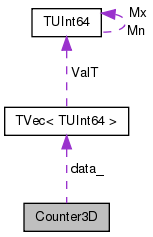[legend]

## Public Member Functions

Counter3D (int m=0, int n=0, int p=0)

const TUInt64operator() (int i, int j, int k) const

TUInt64operator() (int i, int j, int k)

int m ()

int n ()

int p ()

int m_

int n_

int p_

TUInt64V data_

## Detailed Description

Definition at line 46 of file temporalmotifs.h.

## Constructor & Destructor Documentation

 Counter3D::Counter3D ( int m = `0`, int n = `0`, int p = `0` )
inline

Definition at line 48 of file temporalmotifs.h.

References data_, m(), n(), p(), and TVec< TVal, TSizeTy >::PutAll().

48  : m_(m), n_(n), p_(p) {
49  if (m * n * p > 0) {
50  data_ = TUInt64V(m * n * p);
51  data_.PutAll(0);
52  }
53  }
TUInt64V data_
void PutAll(const TVal &Val)
Sets all elements of the vector to value Val.
Definition: ds.h:1229
TVec< TUInt64 > TUInt64V
Definition: ds.h:1595

Here is the call graph for this function: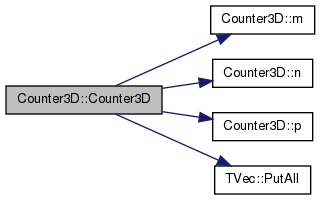## Member Function Documentation

 int Counter3D::m ( )
inline

Definition at line 60 of file temporalmotifs.h.

References m_.

Referenced by Counter3D().

60 { return m_; }

Here is the caller graph for this function: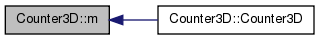int Counter3D::n ( )
inline

Definition at line 61 of file temporalmotifs.h.

References n_.

Referenced by Counter3D().

61 { return n_; }

Here is the caller graph for this function: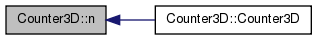const TUInt64& Counter3D::operator() ( int i, int j, int k ) const
inline

Definition at line 54 of file temporalmotifs.h.

References data_, m_, and n_.

54  {
55  return data_[i + j * n_ + k * m_ * n_];
56  }
TUInt64V data_
 TUInt64& Counter3D::operator() ( int i, int j, int k )
inline

Definition at line 57 of file temporalmotifs.h.

References data_, m_, and n_.

57  {
58  return data_[i + j * m_ + k * m_ * n_];
59  }
TUInt64V data_
 int Counter3D::p ( )
inline

Definition at line 62 of file temporalmotifs.h.

References p_.

Referenced by Counter3D().

62 { return p_; }

Here is the caller graph for this function: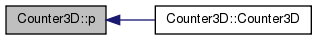## Member Data Documentation

 TUInt64V Counter3D::data_
private

Definition at line 68 of file temporalmotifs.h.

Referenced by Counter3D(), and operator()().

 int Counter3D::m_
private

Definition at line 65 of file temporalmotifs.h.

Referenced by m(), and operator()().

 int Counter3D::n_
private

Definition at line 66 of file temporalmotifs.h.

Referenced by n(), and operator()().

 int Counter3D::p_
private

Definition at line 67 of file temporalmotifs.h.

Referenced by p().

The documentation for this class was generated from the following file: